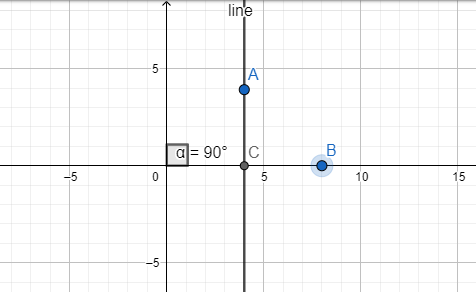QUESTION

# Write the inclination of a line parallel to the y-axis.

Hint: To measure the inclination, we should try to find out the angle made by the line with the x-axis. This angle $\theta$ will be the inclination of the line.

It is given that the line is parallel to the y axis. Now, from the figure below(in which the points A and C lie on the line), we find that angle $\angle ACB$ is a corresponding angle of alpha (as the y-axis and the line are parallel).Also, in the figure, the angle of the line with the x-axis is equal to $\angle ACB$.
Therefore, we find that the slope of the line, given by the angle $\angle ACB$ will be equal to $\alpha ={{90}^{\circ }}$ and thus the inclination of the line w.r.t. the x-axis should be equal to ${{90}^{\circ }}$. Also, as the line and y-axis are parallel to each other, the inclination w.r.t. the y-axis should be zero.
Note: We can also use the equation of the line to find the slope. Two parallel lines will have the same slope. Thus as the y-axis can be represented by the equation $y=mx+c$where $m=\infty$ and thus equivalently as $x=0$, we find that the line parallel to it should also have $m=\infty$and thus its slope should be infinity. The angle with x-axis can be found from the slope using the formula $angle={{\tan }^{-1}}(m)$. And thus $angle={{\tan }^{-1}}\left( \infty \right)={{90}^{\circ }}$ which is the which we had obtained earlier.